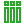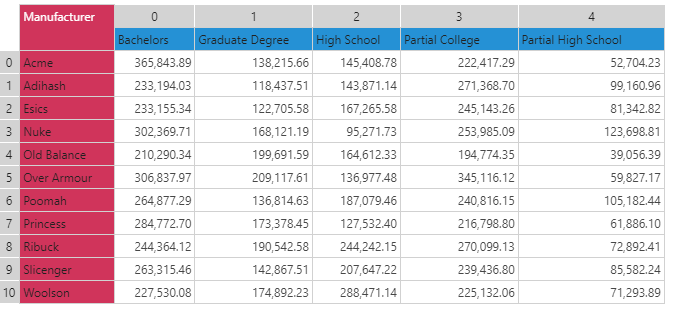#DataSetColumn (PQL)

Returns a list of values found in a column of data in a given query result set.

• Returned Output: Numeric List
• Library: PQL \ Dynamic \ Column
• Version: 2018.00.000
• Compatibility: Any content (regardless of data source) in the relevant parts of the application

#### Syntax

DataSetColumn()

* Click on the function's arguments above for more details on the input values.

• The column number is the zero-based index number of the column of data in the result set.

#### Examples

The examples below use the following example grid.This example returns the list of values from the "Partial College" column (222,417.29 to 225,132.06):

dataSetColumn(3)

This example first finds the column with the highest value using row 0 (lookupMaxColumn). Then, using that index, it returns the list of values from that column (365,843.89 to 227.530.08):

dataSetColumn(lookupMaxColumn(0))